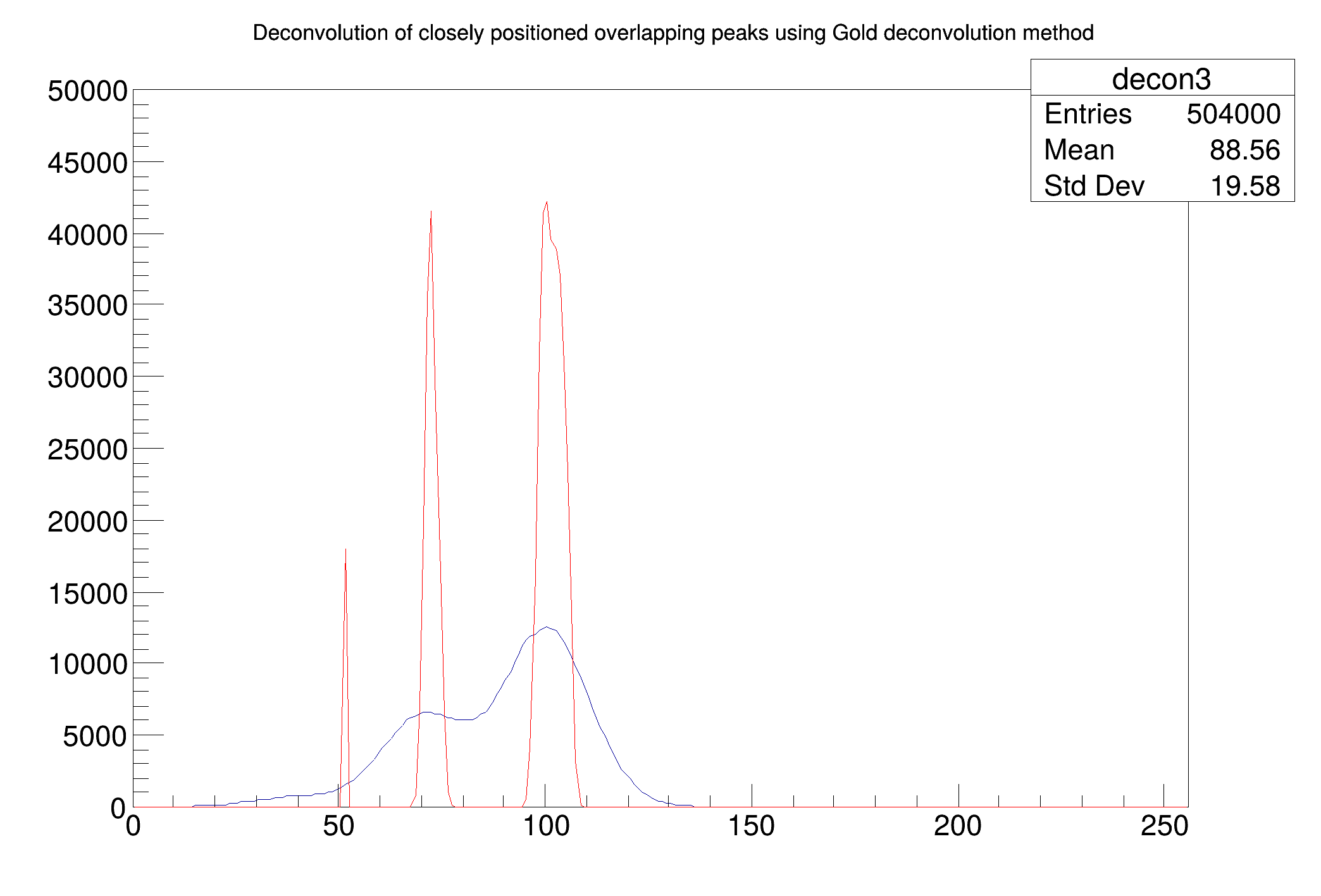ROOT   Reference GuideDeconvolution_wide.C File Reference

## Detailed DescriptionExample to illustrate deconvolution function (class TSpectrum).void Deconvolution_wide() {
Int_t i;
const Int_t nbins = 256;
Double_t xmax = nbins;
Double_t source[nbins];
Double_t response[nbins];
gROOT->ForceStyle();
TString dir = gROOT->GetTutorialDir();
TString file = dir+"/spectrum/TSpectrum.root";
TFile *f = new TFile(file.Data());
TH1F *h = (TH1F*) f->Get("decon3");
h->SetTitle("Deconvolution of closely positioned overlapping peaks using Gold deconvolution method");
TH1F *d = (TH1F*) f->Get("decon_response_wide");
for (i = 0; i < nbins; i++) source[i] = h->GetBinContent(i + 1);
for (i = 0; i < nbins; i++) response[i] = d->GetBinContent(i + 1);
h->SetMaximum(50000);
h->Draw("L");
TSpectrum *s = new TSpectrum();
s->Deconvolution(source,response,256,10000,1,1);
for (i = 0; i < nbins; i++) d->SetBinContent(i + 1,source[i]);
d->SetLineColor(kRed);
d->Draw("SAME L");
}
#define d(i)
Definition: RSha256.hxx:102
#define f(i)
Definition: RSha256.hxx:104
#define h(i)
Definition: RSha256.hxx:106
int Int_t
Definition: RtypesCore.h:45
double Double_t
Definition: RtypesCore.h:59
@ kRed
Definition: Rtypes.h:66
float xmin
Definition: THbookFile.cxx:95
float xmax
Definition: THbookFile.cxx:95
#define gROOT
Definition: TROOT.h:404
A ROOT file is a suite of consecutive data records (TKey instances) with a well defined format.
Definition: TFile.h:54
1-D histogram with a float per channel (see TH1 documentation)}
Definition: TH1.h:574